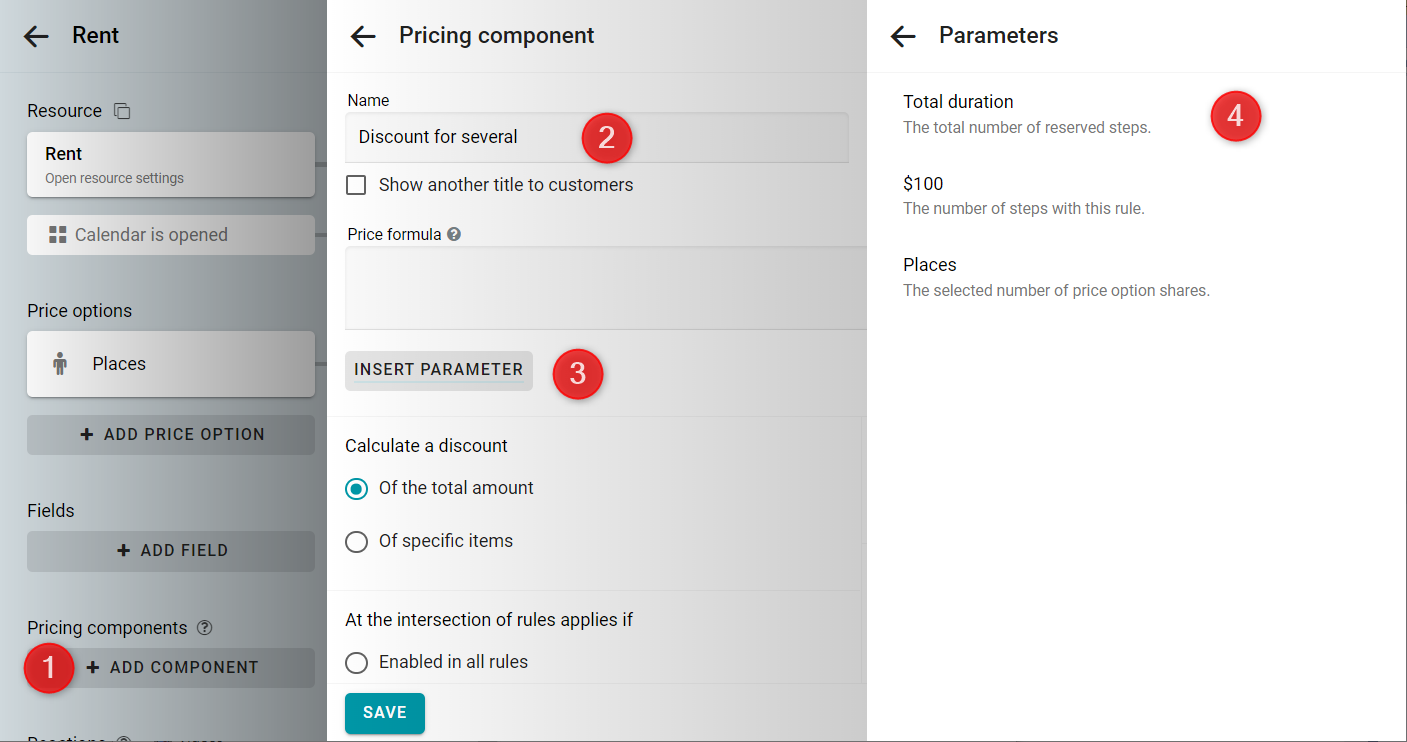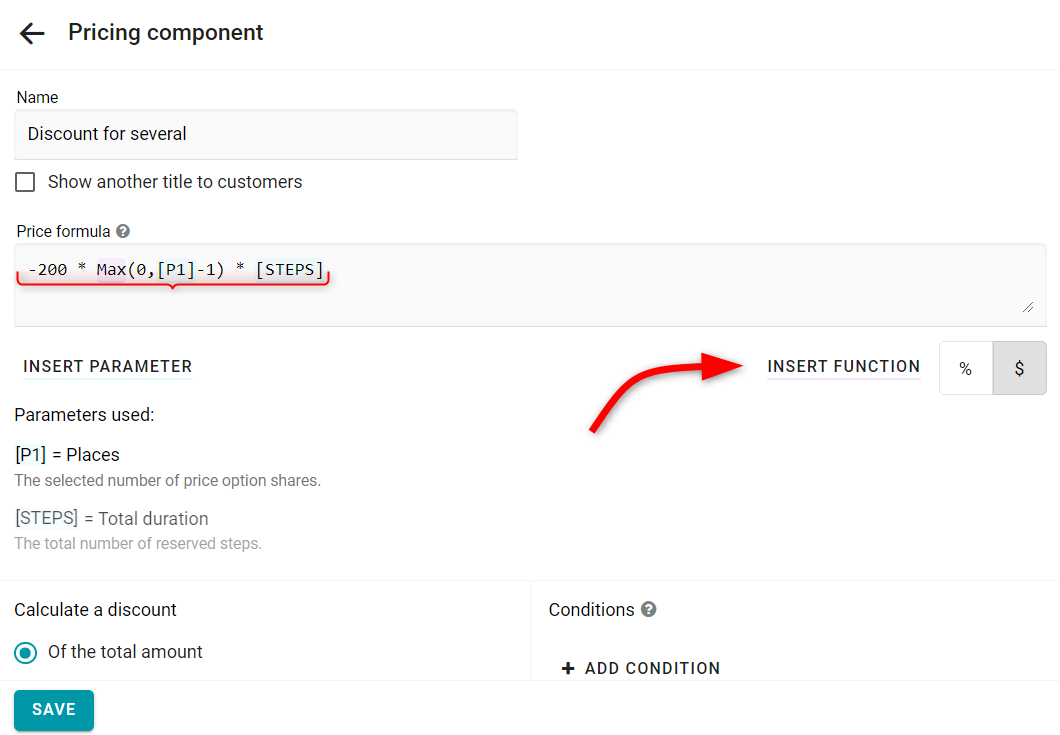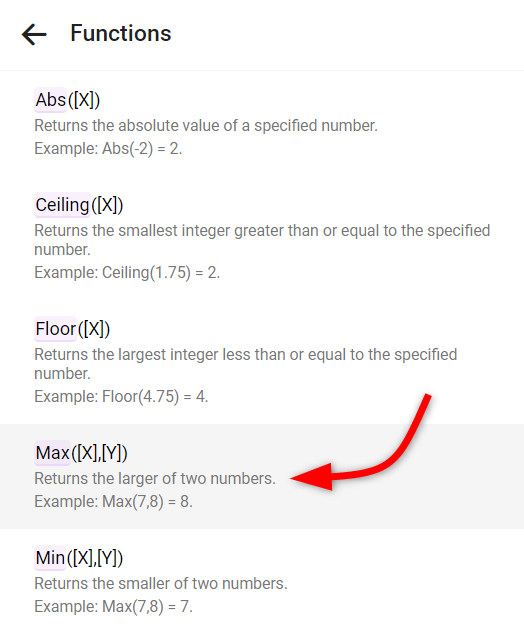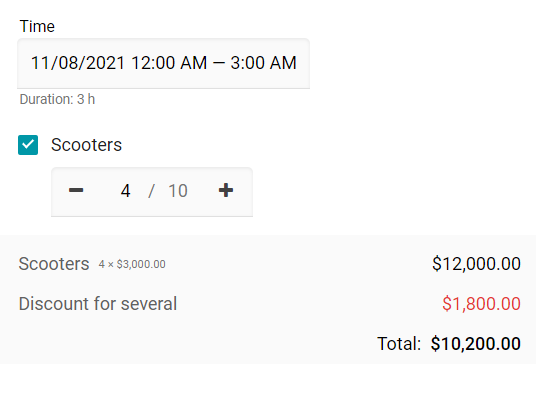arrow_back_iosQuestions
help

# We have a rental price of one scooter \$1000 per hour, but the price of each subsequent scooter is \$800 per hour. How can we get this?

check_circle
Let's say you already have a resource for renting a scooter hourly. The cost of an hour is \$1000.
To offer several scooters cheaper, create a discount for renting more than 1 scooter. Create a pricing component.
Enter a name of the component. Then insert parameters for duration — number of hours [STEPS] and for shares — number of places [P1].Enter the formula. The discount of \$200 is multiplied by the number of scooters over 1. And all this is multiplied by the [STEPS] parameter — the number of reserved hours.
In formula, use the function called Max. It returns the biggest of two numbers. Thus, the expression [P]-1 cannot become less than zero. In order to add a function click on the Insert function button and pick Max from the list of functions.
-200 * Max(0,[P]-1) * [STEPS]Let's check. We will reserve 4 scooters for 3 hours.
The formula calculation: -200 * (4-1) * 3 = \$1800.See the example in action: